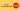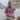# NaN in JavaScript

Posted January 8, 2020Start discussion

The number type in JavaScript holds integers and floats:

``````const integer = 4;
const float = 1.5;

typeof integer; // => 'number'
typeof float;   // => 'number'``````

Plus there are 2 special number values: `Infinity` (a number bigger than any other number) and `NaN` (representing “Not A Number” concept):

``````const infinite = Infinity;
const faulty = NaN;

typeof infinite; // => 'number'
typeof faulty;   // => 'number'``````

While working directly with `NaN` is rare, it can appear surprisingly after a failed operation on numbers.

Let’s take a closer look at `NaN` special value: how to check if a variable has `NaN`, and importantly understand the scenarios that create “Not A Number” values.

## 1. NaN number

The number type in JavaScript is a set of all number values, including “Not A Number”, positive infinity and negative infinity.

“Not A Number” can be accessed using a special expression `NaN`, or as a property of the global object or `Number` function:

``````typeof NaN;        // => 'number'
typeof window.NaN; // => 'number'
typeof Number.NaN; // => 'number'``````

“Not a Number” is a value that does not represent a real number, despite having number type. `NaN` is useful to represent faulty operations on numbers.

For example, multiplying a number with `undefined` is not a valid operation, thus the result is `NaN`:

``1 * undefined;     // => NaN``

Also trying to parse an invalid numeric string like `'Joker'` results in `NaN` too:

``parseInt('Joker', 10); // => NaN``

The section 3. Operations resulting in NaN details into the operations that generate `NaN`.

## 2. Checking for equality with NaN

The interesting property of `NaN` is that it doesn’t equal to any value, even with the `NaN` itself:

``NaN === NaN; // => false``

This behavior is useful to detect if a variable is `NaN`:

``````const someNumber = NaN;

} else {
console.log('Is Not NaN');
}

// logs "Is NaN"``````

`someNumber !== someNumber` expression is `true` only if `someNumber` is `NaN`. Thus the above snippet logs to console `"Is NaN"`.

JavaScript has bult-in functions to detect `NaN`: `isNaN()` and `Number.isNaN()`:

``````isNaN(NaN); // => true
isNaN(1);   // => false

Number.isNaN(NaN); // => true
Number.isNaN(1);   // => false``````

The difference between these functions is that `Number.isNaN()` doesn’t convert its argument to a number:

``````isNaN('Joker12');        // => true
Number.isNaN('Joker12'); // => false``````

`isNaN('Joker12')` converts the argument `'Joker12'` into a number, which is `NaN`. Thus the function returns `true`.

On the other side, `Number.isNaN('Joker12')` checks without conversion if the argument is `NaN`. The function returns `false` because `'Joker12'` doesn’t equal `NaN`.

## 3. Operations resulting in NaN

### 3.1 Parsing numbers

In JavaScript you can transform numeric strings into numbers.

For example, you could easily transform the `'1.5'` string into a `1.5` float number:

``````const numberString = '1.5';
const number = parseFloat(numberString);

number; // => 1.5``````

When the string cannot be converted to a number, the parsing function returns `NaN`: indicating that parsing has failed. Here are some examples:

``````parseFloat('Joker12.5'); // => NaN
parseInt('Joker12', 10); // => NaN
Number('Joker12');       // => NaN``````

When parsing numbers, it’s a good idea to verify if the parsing result is not `NaN`:

``````let inputToParse = 'Invalid10';
let number;

number = parseInt(inputToParse, 10);
if (isNaN(number)) {  number = 0;
}

number; // => 0``````

The parsing of `inputToParse` has failed, thus `parseInt(inputToParse, 10)` returns `NaN`. The condition `if (isNaN(number))` is `true`, and `number` is assigned to `0`.

### 3.2 undefined as an operand

`undefined` used as an operand in arithmetical operations like addition, multiplication, etc. results in `NaN`.

For example:

``````function getFontSize(style) {
return style.fontSize;
}

const fontSize = getFontSize({ size: 16 }) * 2;
const doubledFontSize = fontSize * 2;

doubledFontSize; // => NaN``````

`getFontSize()` is a function that accesses the `fontSize` property from a style object. When invoking `getFontSize({ size: 16 })`, the result is `undefined` (`fontSize` property does not exist in `{ size: 16 }` object).

`fontSize * 2` is evaluated as `undefined * 2`, which results in `NaN`.

“Not A Number” is generated when a missing property or a function returning `undefined` is used as a value in arithmetical operations.

Making sure that `undefined` doesn’t reach arithmetical operations is a good approach to prevent `NaN`. Feel free to check “7 Tips to Handle undefined in JavaScript”.

### 3.3 NaN as an operand

`NaN` value is also generated when an operand in aritemtical operations is `NaN`:

``````1 + NaN; // => NaN
2 * NaN; // => NaN``````

`NaN` spreads across the arithmetical operations:

``````let invalidNumber = 1 * undefined;
let result = 1;
result += invalidNumber; // appendresult *= 2;             // duplicate
result++;                // increment

result; // => NaN``````

Operations on `result` variable are broken after `invalidNumber` value (which has `NaN`) is appended to `result`.

### 3.4 Indeterminate forms

`NaN` value is created when arithmetical operations are in indeterminate forms.

The division of `0 / 0` and `Inifinity / Infinity`:

``````0 / 0;               // => NaN
Infinity / Infinity; // => NaN``````

The multiplication of `0` and `Infinity`:

``0 * Infinity; // => NaN``

Additions of infinite numbers of different signs:

``-Infinity + Infinity; // => NaN``

### 3.5 Invalid arguments of math functions

The square root of negative number:

``````Math.pow(-2, 0.5); // => NaN
(-2) ** 0.5;       // => NaN``````

Or the lograrithm of a negative number:

``Math.log2(-2); // => NaN``

## 4. Conclusion

“Not A Number” concept, expressed in JavaScript with `NaN`, is useful to represent faulty operations on numbers.

`NaN` doesn’t equal to any value, even with `NaN` itself. The recommended way to check if a variable contains `NaN` is to use `Number.isNaN(value)`.

Transforming numeric strings to numbers, when failed, could result in “Not A Number”. It’s a good idea to check whether `parseInt()`, `parseFloat()` or `Number()` don’t return `NaN`.

`undefined` or `NaN` as an operand in arithmetical operations usually result in `NaN`. Correct handling of `undefined` (providing defaults for missing properties) is a good approach to prevent this situation.

Indeterminate forms or invalid arguments for mathematical functions also result in “Not A Number”. But these cases happen rarely.

Here’s my pragmatic advice: “Got `NaN`? Search for `undefined`!”

## Quality posts into your inbox

I regularly publish posts containing:

• Important JavaScript concepts explained in simple words
• Overview of new JavaScript features
• How to use TypeScript and typing
• Software design and good coding practices